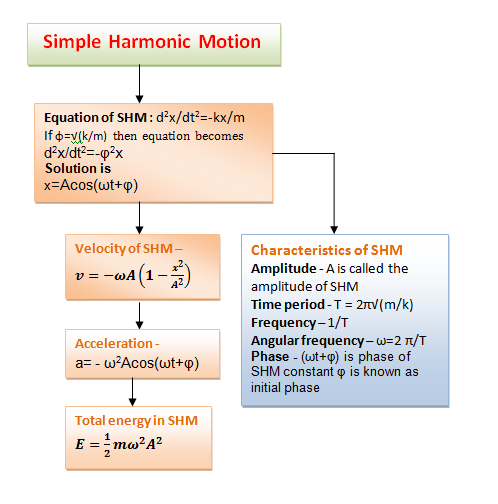# Equation of SHM|Velocity and acceleration|Simple Harmonic Motion(SHM)

### 3. Equation of SHM

• Consider any particle executing SHM with origin as it's equilibrium position under the influence of restoring force F=
• kx , where k is the force constant and x is the displacement of particle from the equilibrium position.
• Now since F= -kx is the restoring force and from Newton's law of motion force is give as F=ma , where m is the mass of the particle moving with acceleration a. Thus acceleration of the particle is
a=F/m
=-kx/m

but we know that acceleration a=dv/dt=d2x/dt2
⇒           d2x/dt2=-kx/m           (1)
This equation 1 is the equation of motion of SHM.
• If we choose a constant φ=√(k/m) then equation 1 would become
d2x/dt2=-φ2x           (2)
• This equation is a differential equation which says that displacement x must be a funcyion of time such that when it's second derivative is calculated the result must be negative constant multiplied by the original function.
• Sine and cosine functions are the functions satisfying above requirement and are listed as follows
x=A sinωt                          (3a)
x=A cosωt                          (3b)
x=A cos(ωt+φ)                     (3c)
each one of equation 3a, 3b and 3c can be submitted on the left hand side of equation 2 and can then be solved for varification.
• Convinently we choose equation 3c i.e., cosine form for representing displacement of particle at any time t from equilibrium position. Thus,
x=A cos(ωt+φ)                     (4)
and A , φ and φ are all constants.
• Fig below shows the displacement vs. time graph for phase φ=0.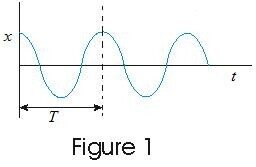### 4. Characterstics of SHM

Here in this section we will learn about physical meaning of quantities like A, T, ω and φ.
(a) Amplitude
• Quantity A is known as amplitude of motion. it is a positive quantity and it's value depends on how oscillations were started.
• Amplitude is the magnitude of maximum value of displacement on either side from the equilibrium position.
• Since maximum and minimum values of any sine and cosine function are +1 and -1 , the maximum and minimum values of x in equation 4 are +A and -A respectively.
• Finally A is called the amplitude of SHM.
(b) Time period
• Time interval during which the oscillation repeats itself is known as time period of oscillations and is denoted by T.
• Since a particle in SHM repeats it's motion in a regular interval T known as time period of oscillation so displacement x of particle should have same value at time t and t+T. Thus,
cos(ωt+φ)=cos(ω(t+T)+φ)
cosine function cos(ωt+φ) will repeat it's value if angle (ωt+φ) is increased by 2π or any of it's multiple. As T is the pime period
(ω(t+T)+φ)=(ωt+φ)+2π
or,          T=2π/ = 2π√(m/k)                (5)
• Equation 5 gives the time period of oscillations.
• Now the frequency of SHM is defined as the numberof complete oscillations per unit time i.e., frequency is reciprocal of time period.
f=1/T = 1/2π(√(k/m))                 (6)
Thus,            ω=2/T = 2f                      (7)
• This quantity ω is called the angular frequency of SHM.
• S.I. unit of T is s (seconds)
f is Hz (hertz)
(c) Phase
• Quantity (ωt+φ) in equation (4) is known as phase of the motion and the constant φ is known as initial phase i.e., phase at time t=0, or phase constant.
• Value of phase constant depends on displacement and velocity of particle at time t=0.
• The knowledge of phase constant enables us to know how far the particle is from equilibrium at time t=0. For example,
If φ=0 then from equation 4
x=A cosωt
that is displacement of oscillating particleis maximum , equal to A at t=0 when the motion was started. Again if φ=/2 then from equation 4
x=A cos(ωt+`/2)
=Asinωt

which means that displacement is zero at t=0.
• Variation of displacement of particle executing SHM is shown below in the fig.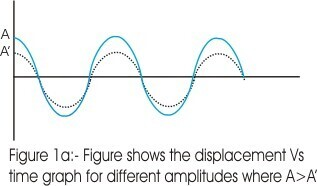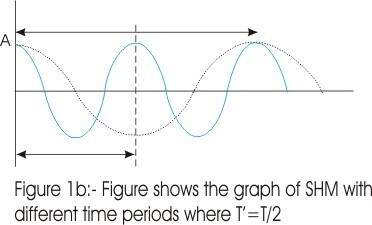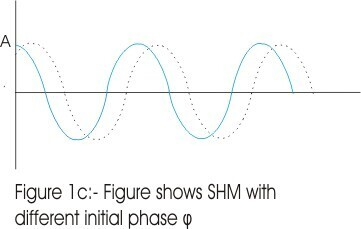### 5.Velocity of SHM

• We know that velocity of a particle is given by
v=dx/dt
• In SHM displacement of particle is given by
x=A cos(ωt+φ)
now differentiating it with respect to t
v=dx/dt= Aω(-sin(ωt+φ))                (8)
• Here in equation 8 quantity Aω is known as velocity amplitude and velocity of oscillating particle varies between the limits ±ω.
• From trignometry we know that
cos2θ + sin2θ=1

A2 sin2(ωt+φ)= A2- A2cos2(ωt+φ)
Or
sin(ωt+φ)=[1-x2/A2]            (9)
putting this in equation 8 we get,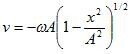• From this equation 10 we notice that when the displacement is maximum i.e. ±A the velocity v=0, because now the oscillator has to return to change it�s direction.
• Figure below shows the variation of velocity with time in SHM with initial phase φ=0.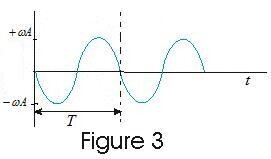### 6. Acceleration of SHM

• Again we know that acceleration of a particle is given by
a=dv/dt
where v is the velocity of particle executing motion.
• In SHM velocity of particle is give by,
v= -ωsin(ωt+φ)
differentiating this we get,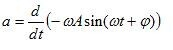or,
a=-ω2Acos(ωt+φ)          (11)
• Equation 11 gives acceleration of particle executing simple harmonic motion and quantity ω2 is called acceleration amplitude and the acceleration of oscillating particle varies betwen the limits ±ω2A.
• Putting equation 4 in 11 we get
a=-ω2x                     (12)
which shows that acceleration is proportional to the displacement but in opposite direction.
• Thus from above equation we can see that when x is maximum (+A or -A), the acceleration is also maximum(-ω2A or +ω2A)but is directed in direction opposite to that of displacement.
• Figure below shows the variation of acceleration of particle in SHM with time having initial phase φ=0.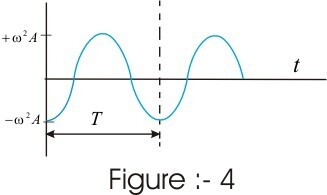## Concept Map for SHM